[BACK]
 Intelligent Automation & Soft ComputingDOI:10.32604/iasc.2020.014388Article

Improved Channel Allocation Scheme for Cognitive Radio Networks

1Department of Computer Science, Shaheed Zulfikar Ali Bhutto Institute of Science and Technology (Islamabad Campus), Islamabad, 44000, Pakistan
2Department of Computer Science, Arid Agriculture University (Burewala Campus), Vehari, 61040, Pakistan
3Department of Software Engineering, Foundation University, Islamabad, 44000, Pakistan
4Department of Computer Engineering, Sungkyul University, Anyang, 430010, South Korea
*Corresponding Author: Sangsoon Lim. Email: lssgood80@gmail.com
Received: 17 September 2020; Accepted: 08 November 2020

Abstract: In recent years, wireless channel optimization technologies witnessed tremendous improvements. In this regard, research for developing wireless spectrum for accommodating a wider range of wireless devices increased. This also helped in resolving spectrum scarcity issues. Cognitive radio (CR) is a type of wireless communication in which a transceiver can intelligently detect which communication channels are being used. To avoid interference, it instantly moves traffic into vacant channels by avoiding the occupied ones. Cognitive Radio (CR) technology showed the potential to deal with the spectrum shortage problem. The spectrum assignment is often considered as a key research challenge in Cognitive Radio Networks (CRNs). In this paper, an evolutionary optimization algorithm is proposed for channel assignment in CRNs. Evolutionary algorithms are inspired by some type of biological evolution technique. In the proposed technology we used Particle Swarm Optimization (PSO). The resulting algorithm is called differential evolution-based particle swarm optimization with the repair process (DEPSO-RP). Moreover, a repair process is introduced to remove conflicts among secondary users (SUs) to increase the spectrum in CRNs. The performance of DEPSO-RP spectrum assignment algorithm has been evaluated by extensive simulations. The proposed spectrum assignment algorithm showed better performance regarding channel assignment in comparison with other existing algorithms in the literature.

Keywords: Evolutionary optimization; cognitive radio network; channel assignment; particle swarm optimization

1  Introduction

The recent increase witnessed in the demand for wireless gadgets in daily communications for example cellular phones, wireless sensors, and tablets has risen exponentially [1,2]. It is due to the enormous usage of IT-based technologies such as cloud computing and IoT, where different smart devices securely communicate using a standard format of communication [3,4]. The enormous usage of devices increased the data rate demands many folds. The present spectrum allocation is fixed and unable to fulfill the growing demands of data transfer rates. This has promoted the development of next-generation networks which are known as the fifth-generation networks. Cognitive Radio communication is an important feature of fifth-generation networks. In cognitive radio communication, the wireless spectrum is dynamically accessed for exploiting the underutilized spectrum .

Wireless spectrum is of fundamental importance when it comes to efficient network resource utilization. The licensed users known as primary users (PUs), do not utilize the spectrum all the time. The unused spectrum, therefore, can be utilized for different applications. Unlicensed users are known as secondary users . Cognitive communication is a technology that continuously monitors the spectrum both in time and frequency domains. Moreover, the users with a license to utilize the spectrum can use it when the PUs are idle. The SUs utilize free spectrum slots when the PUs is not available. Similarly, the free spectrum slots can also be utilized when SUs transmission power is below the interference threshold allowed by the PUs . The methodology of exploring new spectrum locations is called dynamic spectrum access technology (DSA) . The spectrum efficiency is enhanced by using optimization techniques with DSA . Although the spectrum efficiency is improved by using DSA there is always a tradeoff between data rate and interference  in CRNs. The resource optimization in CRNs is considered as a nondeterministic polynomial problem . Efficient spectrum allocation and minimizing the interference is considered as an optimization problem. There are many optimization techniques proposed to deal with this problem such as convex optimization, non-convex optimization, relaxation, and other techniques . In CRNs, the resource allocation problem is an important challenge and is widely studied in the literature . The spectrum auction-based allocation techniques in CRNs is discussed in Zhao et al. .

Traditional mathematical optimization techniques such as the Lagrange multipliers requiring derivatives have difficulty in handling discrete variables . The EC algorithms are inspired by the biological evolution of species . In Ali et al. , authors used evolutionary algorithms for power optimization in the internet of things applications whereas the ant colony optimization is studied for spectrum assignment in CRNs . In Pang et al. [21,22], the particle swarm optimization (PSO) algorithms are used to optimize the spectrum resources. A compact prefix tree based metaheuristic algorithm is presented in Jin et al.  for spectrum allocation. In Han et al. , a relay cognitive radio network is presented. The graph method is studied for spectrum optimization in Peng et al. . The results demonstrate that the proposed methodology has a slow convergence rate. The fuzzy-based approach is studied for spectrum allocation in Koroupi et al. . The spectrum allocation for conflicting SUs is not addressed and is often considered a challenge in research. Although there are many spectrum assignment algorithms, comprehensive literature on efficient spectrum assignment for conflicting SUs is needed.

In this paper, we present an improved hybrid algorithm for channel assignment in CRNs. Moreover, a repair process is introduced to improve spectrum utilization for those SUs which are accessing the same channel. The simulation results are analyzed and compared with other evolutionary algorithms in the literature. The objectives of this study are:

•   Maximizes the network utilization

•   Minimizes the interference among SUs

Section II explains the system model. Section III provides our proposed method. Section IV discusses the comparisons between the proposed method and four other evolutionary methods under the same benchmark constraints. The paper is concluded in Section V.

2  System Model

We consider a CRN model consists of X number of PUs and I numbers of SUs that are randomly placed as shown in Fig. 1. The PUs has M channels for their communication. These channels can be utilized for SUs communication provided that PUs is not using these channels or SUs are far away from PUs.

The proposed system model is illustrated in Fig. 1. Here, dp represents the protection region of each PU, whereas di represents the transmission range of each SU. We also considered orthogonal frequency division multiplexing in the proposed model. . In Fig. 1, it can be seen that the SUs outside the PUs protection region dp can communicate provided that their transmission range remains in the allowed limit.

The SU-I transmission range is overlapping with protection regions of PU-I, therefore, the SU is not allowed to communicate. It is noteworthy that the secondary user’s II, III, and IV are far away from PUs so these users can communicate with each other. Although, SU-V and SU-VI are far away from PUs their transmission range is overlapping so they also do not qualify to access the free spectrum.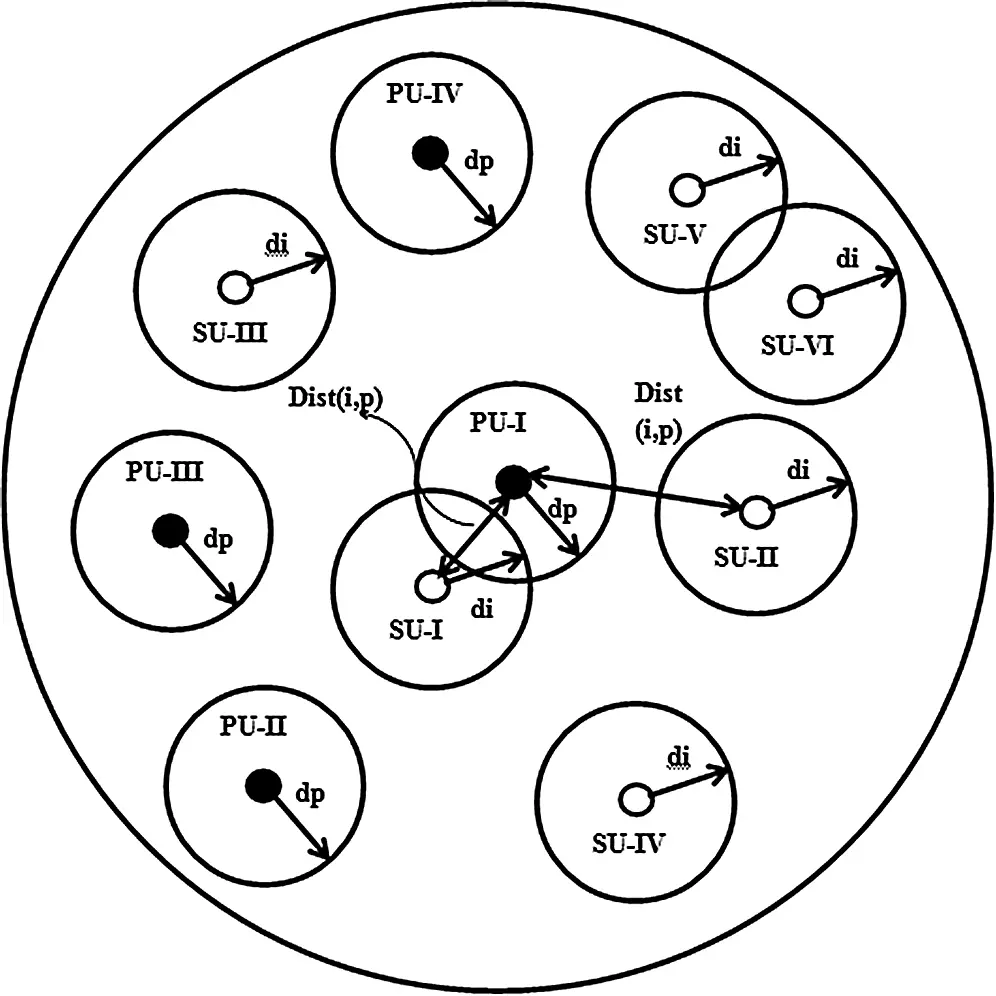Figure 1: System model

The SUi can utilize the channel m of the nearest PUp as long as Dist(i,m)-d(p,m)≥d(i,m), where d(i,m) represents the transmission range of SUi on channel m, Dist(i,p) is the distance between SUi and PUp, and d(p,m) is the transmission range of PUp using channel m.

First, let us define a binary channel availability matrix L(I×M) in which each element l(i,m)=1 indicates that SUi can use channel m only if its transmission range is below the allowed limit, otherwise l(i,m)=0. Let A(I×M) represents the binary channel assignment matrix. In this matrix, if a(i,m)=1then channel m is assigned to SUi; otherwise a(i,m)=0. The channel assignment must satisfy interference constraint C={ci,k,m} which is based on distances between SUs. If two SUs i and k are competing for the same channel m, the channel accessing criteria isIn Eq. (1), ci,k,m=0 represents SUi and SUk are not interfering with each other. It indicates that SUi and k are far away from each other. Conversely, if ci,k,m=1, only one SU can use the channel m. The data rate ri,m of SUi using the channel, m is based on signal to noise γi,m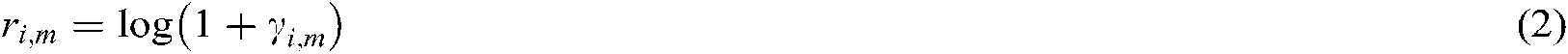The spectrum utilization of SUs in a CRN is defined by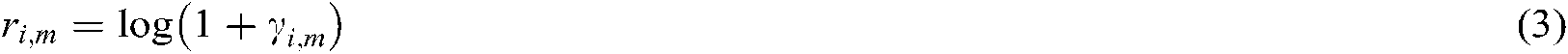Eq. (3) shows that the spectrum utilization R is based on the reward sum of all SUs I over M channels. The objective function to maximize the spectrum utilization is defined by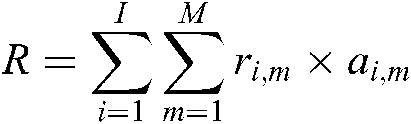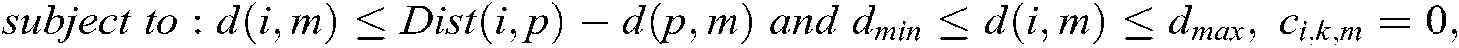Eq. (4) shows that spectrum utilization of SUi using channel m is maximized subject to fulfill the above constraints. In Eq (4), Cmax corresponds to the upper limit of channels that an SUi can use. In Eq. (4), dmin and dmax represents the minimum and maximum transmission range allowed to SUs. If the SU transmission range is di<dmin , the SU is not qualifying to access free channels because its transmission range is below the transmission threshold. Similarly, if di>dmax, the SU is not allowed to communicate because large transmission range di may result in more interference with neighboring SUs. Spectrum utilization mentioned in Eq. (4) is a multi-constraint problem.

3  Proposed DEPSO Channel Assignment Algorithm

In DEPSO, the PSO is integrated with the differential evolution (DE) algorithm to form a hybrid approach. PSO is a population-based algorithm . The population is represented by a swarm of particles where particles represent the solution to the problem. The velocity and position of each particle are the main parameters of PSO. The movement of every particle in the swarm is guided by its own best previous position found by itself and that is found by neighboring particles . Particle position xi of SUi is adjusted using PSOIn Eq. (5), vi represents the velocity component of SUi and is updated using Eq. (6).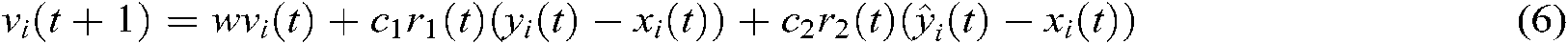where yi (t) is own best position of particle i,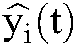is the neighboring best position of particle i, w is the inertia weight, c1 and c2 are amplifying coefficients, and r1,r2∈U(0,1).

DE is a population-based evolutionary algorithm . In DE, the position is updated stochastically as a weighted difference between randomly selected individuals. For each parent node xi (t), select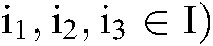where the population size is I, and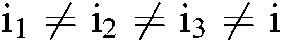. Let x1, x2, and x3 are randomly selected positions of particles from the population. The position of SUi is updated based on DE and is expressed mathematically as follows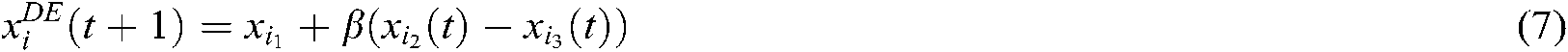The position update as followsIn Eq. (8), position xi(t+1) of the particle, i is updated from xiPSO facilitating exploitation while for a proportion of the population is updated by xiDE facilitating exploration. In Eq. (8), U(0,1) representing uniform distribution between 0 and 1, N(0.7,0.2) denoting normal distribution with mean 0.7 and variance 0.2. These values are selected after extensive simulation results to obtain better results. The PSO algorithm converges very quickly due to the inclusion of personal best experience and neighbor’s best experience. The consideration of past best results is called exploitation. On the other hand, DE converges to the actual optimal value very closely due to its large search space (exploration). This paper presents a hybrid algorithm, which combines exploitation concepts of PSO and explores concepts of DE. The resulting algorithm referred to as the DEPSO provides a good balance between exploitation. The particles will eventually converge to the optimal position. The general description of the DEPSO-RP spectrum assignment algorithm is given in Fig. 2. Now we analyze the DEPSO algorithm for channel assignment in CRN. Eq. (1) is considered as the fitness function.

One of the important challenges is mapping between problem and solution. Here, particles correspond to SUs, and positions of particles correspond to the channels accessing by SUs as shown in Fig. 3. In Figs. 3 and 1 represents that the channel is occupied and 0 represents that channels are unoccupied and free to use.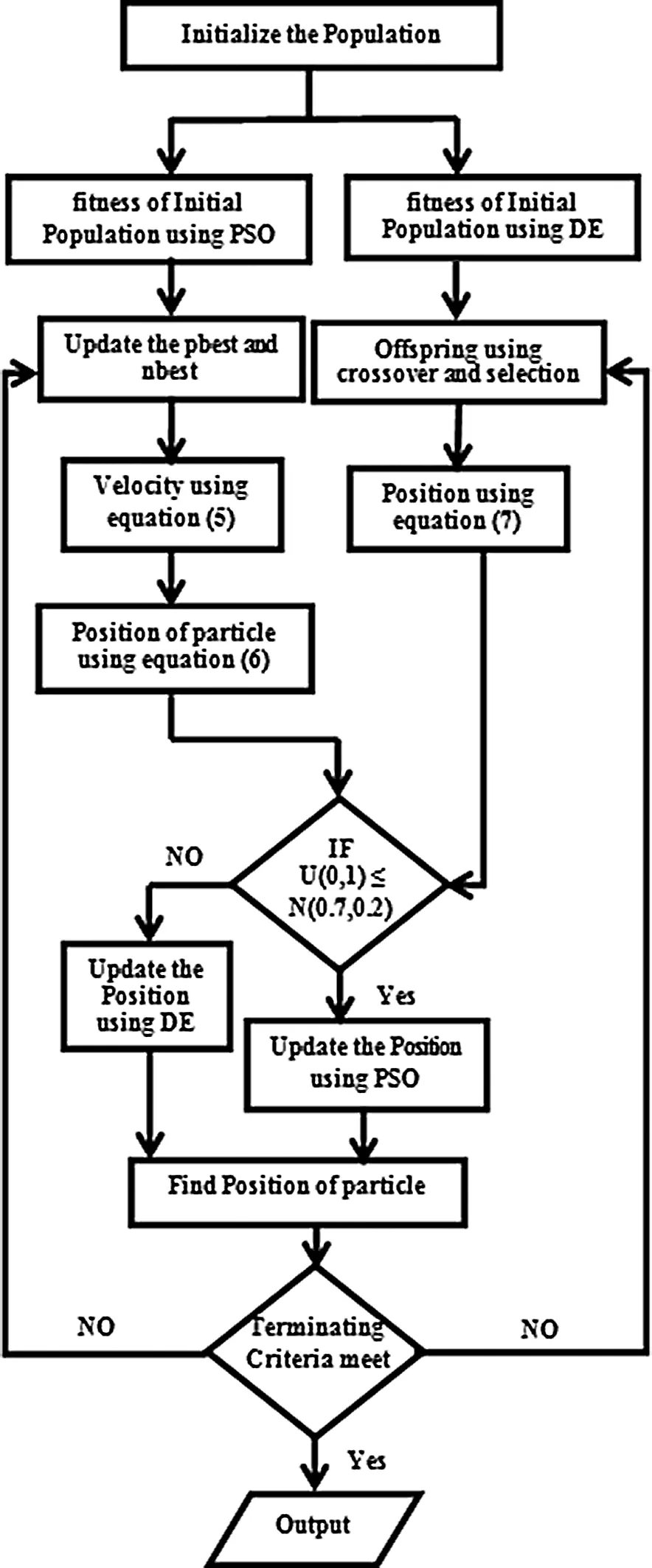Figure 2: Flow chart of DEPSO-RP algorithmFigure 3: Channel assignment using DEPSO-RP

In the proposed DEPSO-RP spectrum assignment algorithm, the velocity v_im represents the movement of the particle SUi using channel m from the current slot to the next slot. It is assumed that SUs can move three slots forward or backward. For example, if a SU currently connected with the 3rd channel, in next-generation, that SU can join channels 2, 4, or 6.

The algorithm starts to generate a random solution according to the interference constraint defined in section II. The algorithm sequentially assigns the available channels to SUs. The next step is to ensure that each feasible channel should be assigned to only one SU at a given time instant. Fig. 4a shows an example of the repair process. In Fig. 4a, SU2 and SU3 are occupying the same channel at the same time. This means that channel allocation violates the interference constraint mentioned in Eq. (1). Discarding a SU can reduce solution space specifically when a low proportion of the population is feasible.Figure 4: Channel assignment to SUs. (a) The clash between SU2 and SU3. (b) Adjusting positions using the repair process

The repair process will be performed for SUs to replace their positions. As shown in Fig. 4b, SU 3 moves to the 5th slot so that there is no conflict now between these two SUs. By doing so, the spectrum utilization for SUs is improved.

4  Results and Discussion

Simulations are performed to evaluate the efficiency of the proposed solution. The parameter values for the simulations are represented in Tab. 1. Here, a CRN consists of X number of randomly placed PUs and I SUs in the proposed circular area. The results of the proposed algorithm are compared with other evolutionary algorithms such as Particle swarm optimization with repair process (PSO-RP), Differential evolution with repair process (DE-RP), and with randomly generated population (RAND). The proposed channel assignment algorithm is analyzed for different ranges of SUs, PUs, and available channels. Moreover, the performance of spectrum utilization is compared for different ranges of cycles and transmission ranges of SUs.

Table 1: Different parameter values used in the experiment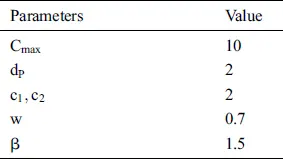The performance of spectrum utilization against a varying number of channels is analyzed and is shown in Fig. 5. Here, simulations are done considering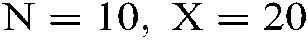. As can be seen from Fig. 5, the performance of DEPSO-RP is superior as compared to other algorithms due to the inclusion of the repair process which increases spectrum utility significantly. The DEPSO-RP algorithm has both exploration and exploitation capabilities due to which it performs better.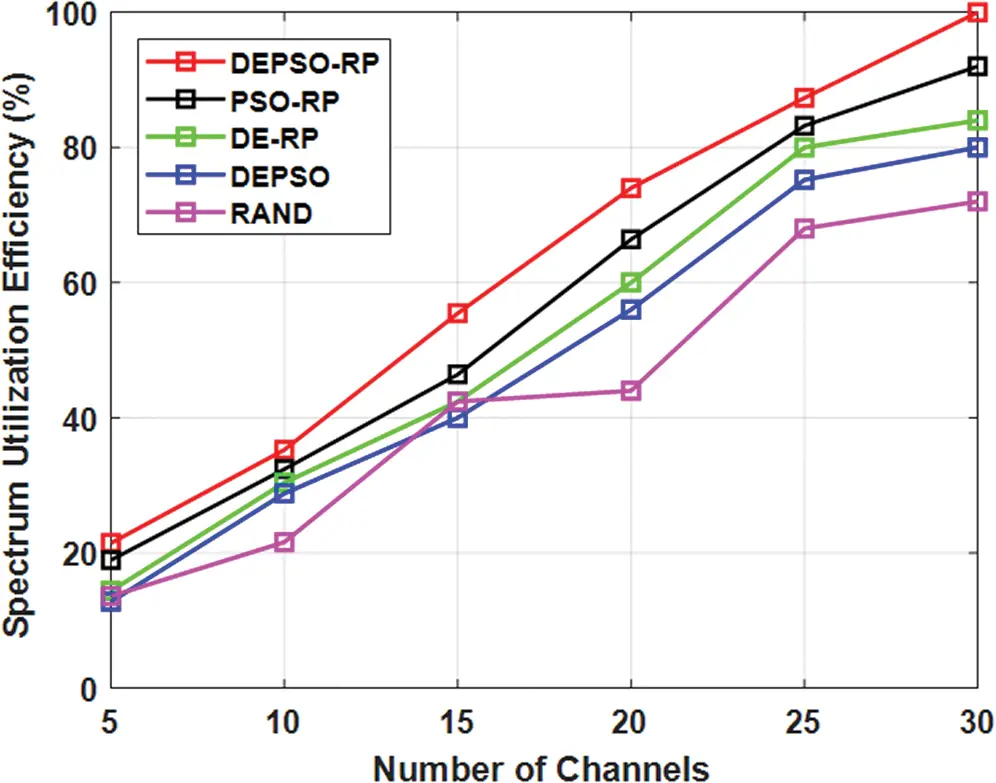Figure 5: Spectrum Utilization against a varying number of channels

If the number of SUs is increased in a fixed area, spectrum utilization would surely be reduced. The performance of DEPSO-RP for spectrum utilization is analyzed against the increasing number of SUs as shown in Fig. 6. Moreover, the results of DEPSO-RP are evaluated with other similar algorithms. increasing the SUs in the same fixed area creates additional interference constraints. The simulations are conducted for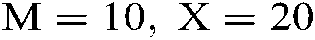. The results of Fig. 6 show that the performance of spectrum utilization is reduced with the increasing number of SUs. The DEPSO-RP algorithm performs remarkably well due to its blend of both exploration and exploitation capability.

Similarly, the number of PUs would reduce the chances of SUs for obtaining channels. The results are shown in Fig. 7 for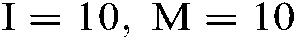. The results of Fig. 7 showed that DEPSO-RP outperforms the other understudied algorithms.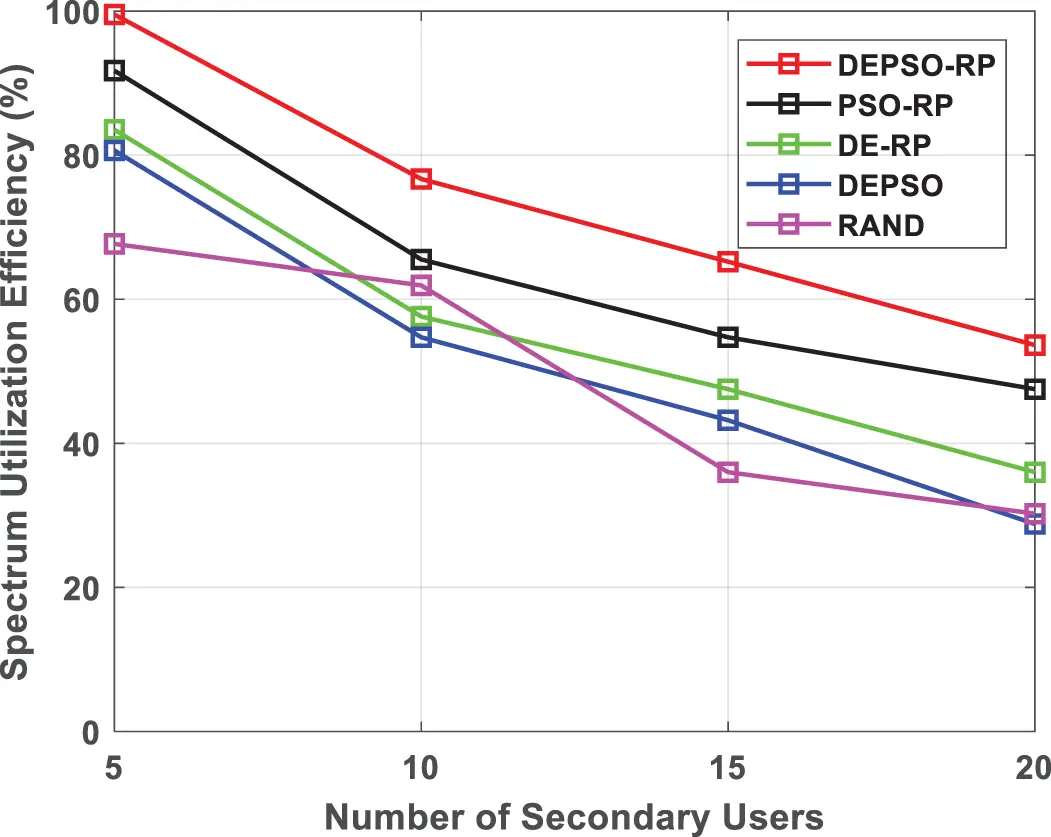Figure 6: Spectrum utilization against a varying number of SUs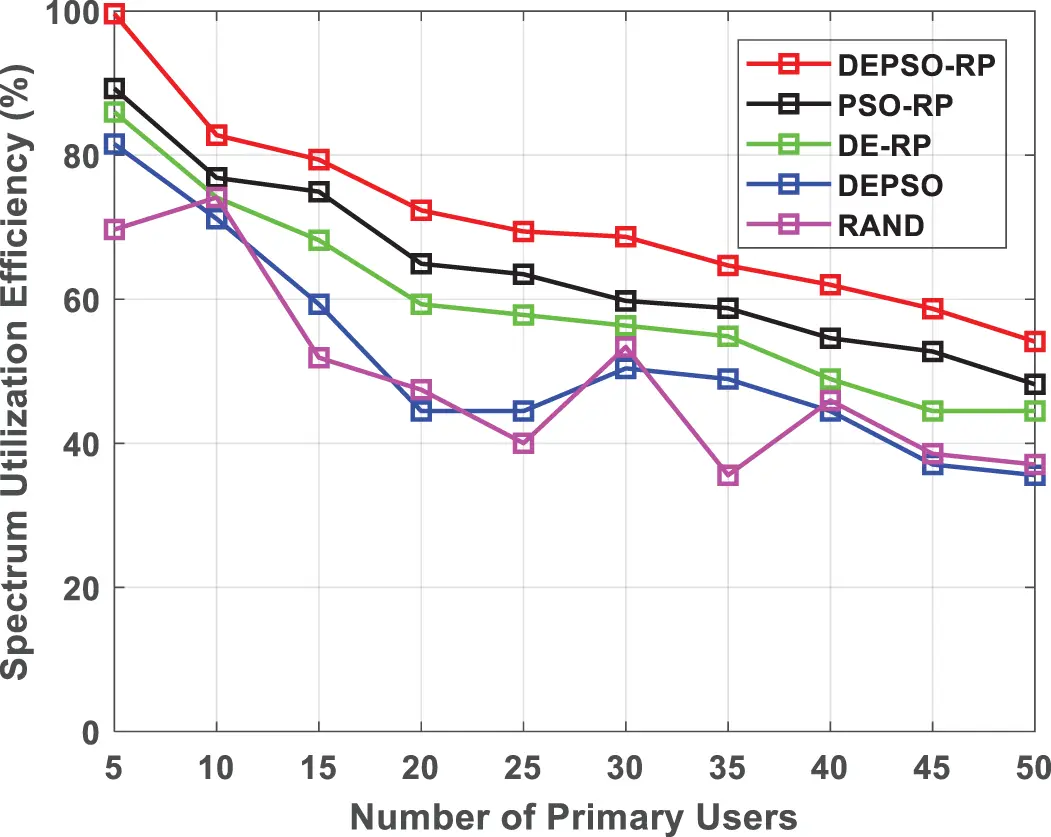Figure 7: Spectrum Utilization against varying PUs

The faraway SUs from PUs can improve their spectrum utilization by increasing their transmission range d_max. However, this increasing transmission power can cause more interference to adjacent SUs. So it depends on SUs location whether to increase their transmission range or not beyond a certain level. In Fig. 8, the performance of spectrum utilization is analyzed against a varying range of dmax. The results show that varying transmission range changes the value of spectrum utilization significantly.

In Fig. 9, the transmission range of all SUs is fixed so that all SUs get the uniform reward. The performance of spectrum utilization against different values ofdmin=dmax is evaluated in Fig. 9. The result shows that the spectrum utilization attains the maximum value at dmin=dmax=5. As shown from the results, again DEPSO-RP outclasses the other understudied channel assignment algorithms. The simulations are conducted on a core-i5 processor with 6 GB of RAM.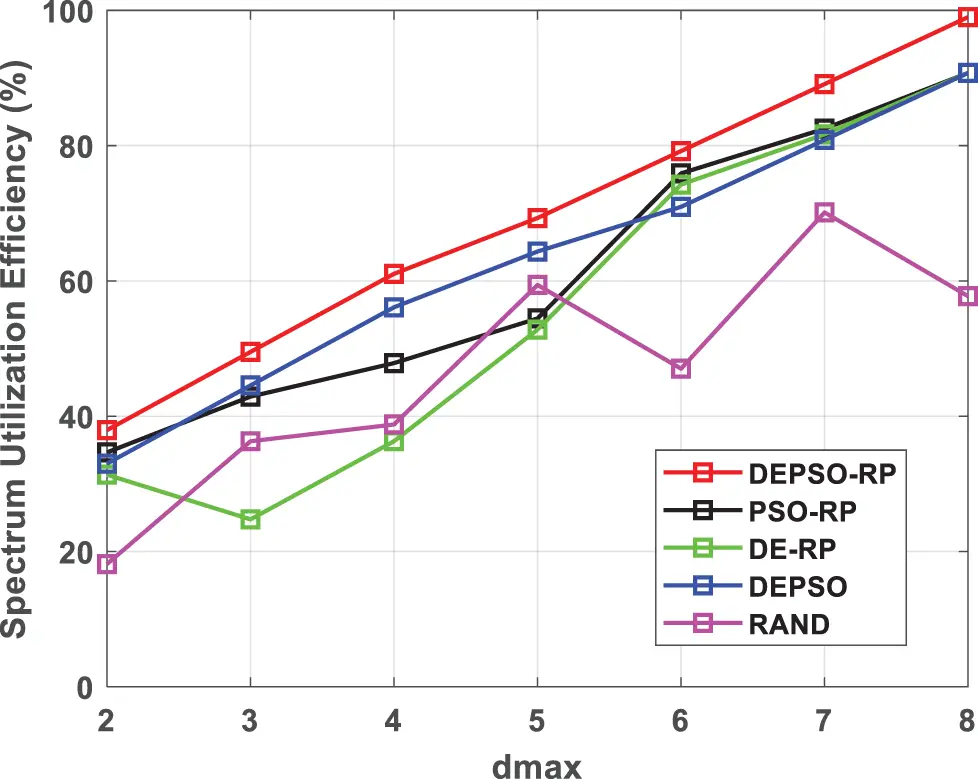Figure 8: Spectrum utilization against a varying range of d_maxFigure 9: Spectrum utilization against varying maximal transmission range for SUs (dmax=dmin)

The above results demonstrate that the DEPSO-RP algorithm converged to the best solutions much faster than the other understudied algorithms. The performance of DEPSO-RP is improved due to two reasons. First, it combines the good characteristics of PSO and DE. Second, the repair process improved the spectrum utilization for conflicting SUs.

5  Conclusion

The recent demand for the availability of wireless spectrum has been increased dramatically. It is due to the vast-scale invention of various wireless technologies. Spectrum allocation is a key research problem in CRNs. In this paper, a repair process-based channel assignment mechanism is presented. It aims to optimize spectrum utilization. The performance of the proposed algorithm is evaluated against different network topologies with varying numbers of PUs, SUs, and channels. Moreover, the results of the proposed solution are also compared with other state-of-the-art evolutionary algorithms. Simulation results demonstrate that the proposed DEPSO-RP spectrum assignment algorithm has improved CRN spectrum utilization.

Funding Statement: This research was supported by the National Research Foundation of Korea (NRF) grant funded by the Korea government (MSIT) (No. NRF-2018R1C1B5038818).

Conflicts of Interest: The authors declare that they have no conflicts of interest to report regarding the present study.

## References

1. C. Zhu, V. C. M. Leung, L. Shu and E. C. H. Ngai. (2015). “Green internet of things for smart world,” IEEE Access, vol. 3, pp. 2151–2162.
2. A. Venckauskas, V. Stuikys, R. Damasevicius and N. Jusas. (2016). “Modelling of internet of things units for estimating security-energy-performance relationships for quality of service and environment awareness,” Security and Communication Networks, vol. 9, no. 16, pp. 3324–3339.
3. D. Plonis, A. Katkevičius, A. Gurskas, V. Urbanavičius, R. Maskeliūnas et al. (2020). , “Prediction of meander delay system parameters for internet-of-things devices using pareto-optimal artificial neural network and multiple linear regression,” IEEE Access, vol. 8, pp. 39525–39535.
4. W. Wei, X. Xia, M. Wozniak, X. Fan, R. Damaševičius et al. (2019). , “Multi-sink distributed power control algorithm for cyber-physical-systems in coal mine tunnels,” Computer Networks, vol. 161, pp. 210–219.
5. S. Chen and J. Zhao. (2014). “The requirements, challenges, and technologies for 5G of terrestrial mobile telecommunication,” IEEE Communications Magazine, vol. 52, no. 5, pp. 36–43.
6. I. F. Akyildiz, W. Y. Lee, M. C. Vuran and S. Mohanty. (2006). “Next generation/dynamic spectrum access/cognitive radio wireless networks: A survey,” Computer Networks, vol. 50, no. 13, pp. 2127–2159.
7. S. Haykin. (2005). “Cognitive radio: Brain-empowered wireless communications,” IEEE Journal on Selected Areas in Communications, vol. 23, no. 2, pp. 201–220.
8. W. Y. Lee and I. Akyildiz. (2012). “Spectrum-aware mobility management in cognitive radio cellular networks,” IEEE Transactions on Mobile Computing, vol. 11, no. 4, pp. 529–542.
9. Z. Liu, B. Liu and C. W. Chen. (2017). “Buffer-aware resource allocation scheme with energy efficiency and QoS effectiveness in wireless body area networks,” IEEE Access, vol. 5, pp. 20763–20776.
10. G. Yu, L. Xu, D. Feng, R. Yin, G. Y. Li et al. (2014). , “Joint mode selection and resource allocation for device-to-device communications,” IEEE Transactions on Communications, vol. 62, no. 11, pp. 3814–3824.
11. J. Yu, M. Li, Y. Wang and G. He. (2014). “A decomposition method for large scale box constrained optimization,” Applied Mathematics and Computation, vol. 231, pp. 9–15.
12. H. Mei, K. Wang and K. Yang. (2017). “Multi-layer cloud-RAN with cooperative resource allocations for low-latency computing and communication services,” IEEE Access, vol. 5, pp. 19023–19032.
13. F. Zhao, L. Wei and H. Chen. (2016). “Optimal time allocation for wireless information and power transfer in wireless powered communication systems,” IEEE Transactions on Vehicular Technology, vol. 65, no. 3, pp. 1830–1835.
14. S. Latif, S. Akraam and M. A. Saleem. (2017). “Joint optimization of interference and cost in cognitive radio heterogeneous network using fuzzy logic powered ants,” Wireless Communications and Mobile Computing, vol. 2017, pp. 1–11.
15. K. Yang, L. Wang, S. Wang and X. Zhang. (2017). “Optimization of resource allocation and user association for energy efficiency in future wireless networks,” IEEE Access, vol. 5, pp. 16469–16477.
16. F. Zhao, H. Nie and H. Chen. (2017). “Group buying spectrum auction algorithm for fractional frequency reuse cognitive cellular systems,” Ad Hoc Networks, vol. 58, pp. 239–246.
17. C. Han, T. Feng, G. He and T. Guo. (2013). “Parallel variable distribution algorithm for constrained optimization with non monotone technique,” Journal of Applied Mathematics.
18. M. R. Bonyadiand and D. C. Reutens. (2019). “Optimal-Margin evolutionary classifier,” IEEE Transactions on Evolutionary Computation, vol. 23, no. 5, pp. 885–898.
19. H. M. Ali, W. Ejaz, D. C. Lee and I. M. Khater. (2019). “Optimising the power using firework-based evolutionary algorithms for emerging IoT applications,” IET Networks, vol. 8, no. 1, pp. 15–31.
20. Q. He and P. Zhang. (2012). “Dynamic channel assignment using ant colony optimization for cognitive radio networks,” in Proc. VTC’12, Montreal, Quebec, Canada, pp. 1–5.
21. S. Pang, T. Li, F. Dai and M. Yu. (2013). “Particle swarm optimization algorithm for multi-salesman problem with time and capacity constraints,” Applied Mathematics and Information Sciences, vol. 7, no. 6, pp. 2439–2444.
22. E. Vega-Alvarado, E. A. Portilla-Flores, M. B. Calva-Yáñez, G. Sepúlveda-Cervantes, J. A. Aponte-Rodríguez et al. (2017). , “Hybrid metaheuristic for designing an end effector as a constrained optimization problem,” IEEE Access, vol. 5, pp. 6002–6014.
23. H. Jin, A. A. Abbasi and S. Wu. (2017). “Pathfinder: Application-aware distributed path computation in clouds,” International Journal of Parallel Programming, vol. 45, no. 6, pp. 1273–1284.
24. L. Han, J. Mu, W. Wang and B. Zhang. (2014). “Optimization of relay placement and power allocation for decode-and-forward cooperative relaying over correlated shadowed fading channels,” EURASIP Journal of Wireless Communication Networks, vol. 1, pp. 1–41.
25. C. Peng, H. Zheng and B. Zhao. (2006). “Utilization and fairness in spectrum assignment for opportunistic spectrum access,” Mobile Networks and Applications, vol. 11, no. 4, pp. 555–576.
26. F. Koroupi, H. Salehinejad and S. Talebi. (2013). “Spectrum assignment in cognitive radio networks using fuzzy logic empowered ants,” Iranian Journal of Fuzzy Systems, vol. 10, no. 6, pp. 1–19.
27. A. P. Engelbrecht. (2007). Computational intelligence: An introduction. John Wiley & Sons.
28. E. S. Peer, F. van den Bergh and A. P. Engelbrecht. (2003). “Using neighborhoods with the guaranteed convergence PSO,” in Proc. SIS’03, Indianapolis, Indiana, USA, pp. 235–242.This work is licensed under a Creative Commons Attribution 4.0 International License, which permits unrestricted use, distribution, and reproduction in any medium, provided the original work is properly cited.